# Vue+Element-UI 中 el-table 动态合并单元格 :span-method 方法

el-table官方提供了合并单元格的方法与返回格式 如下: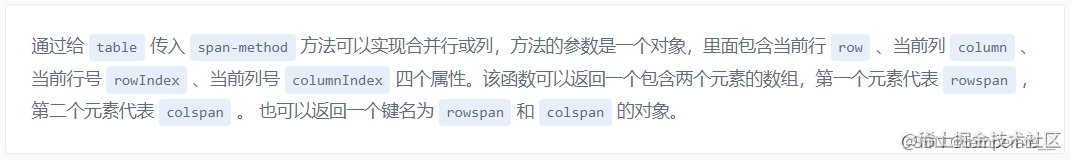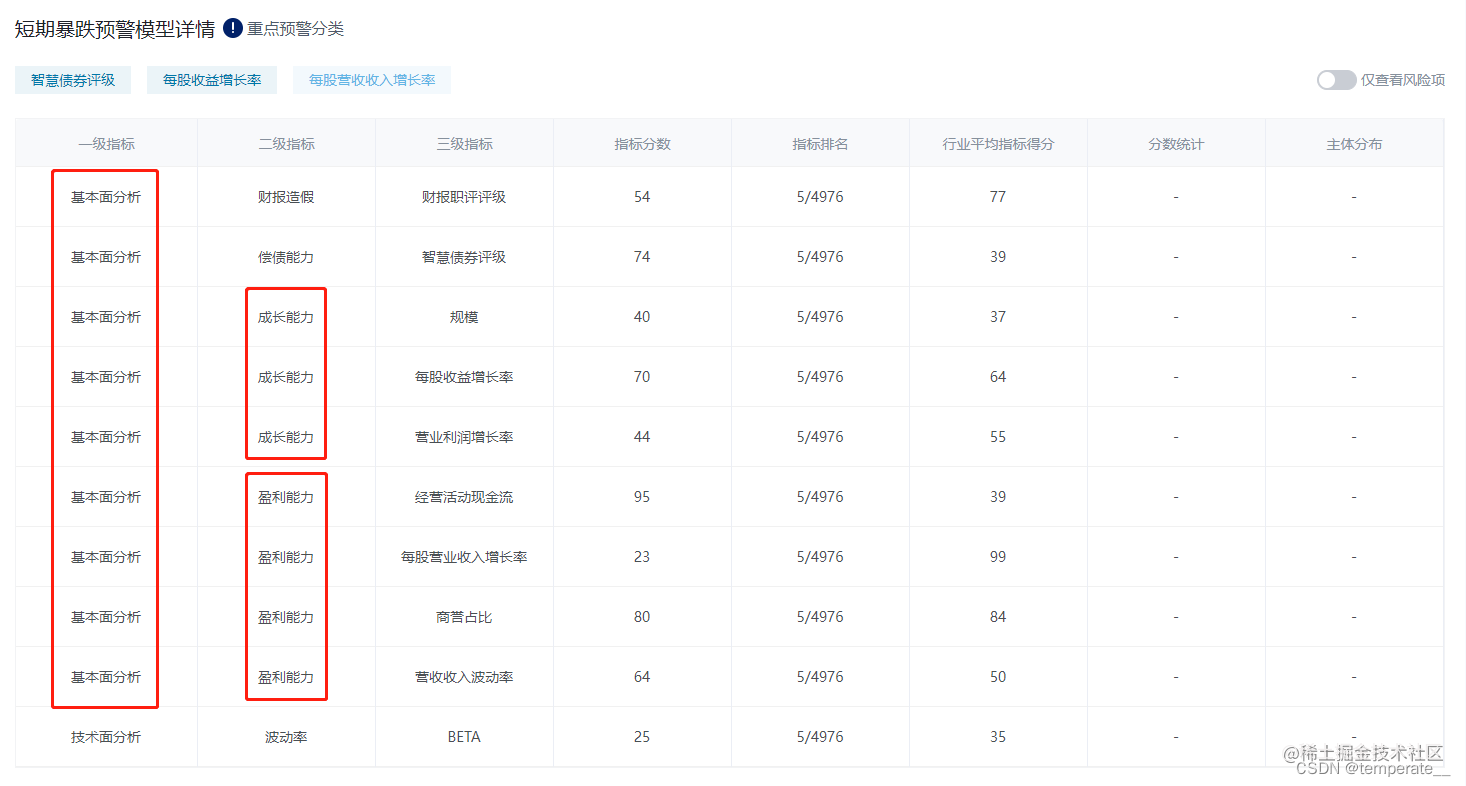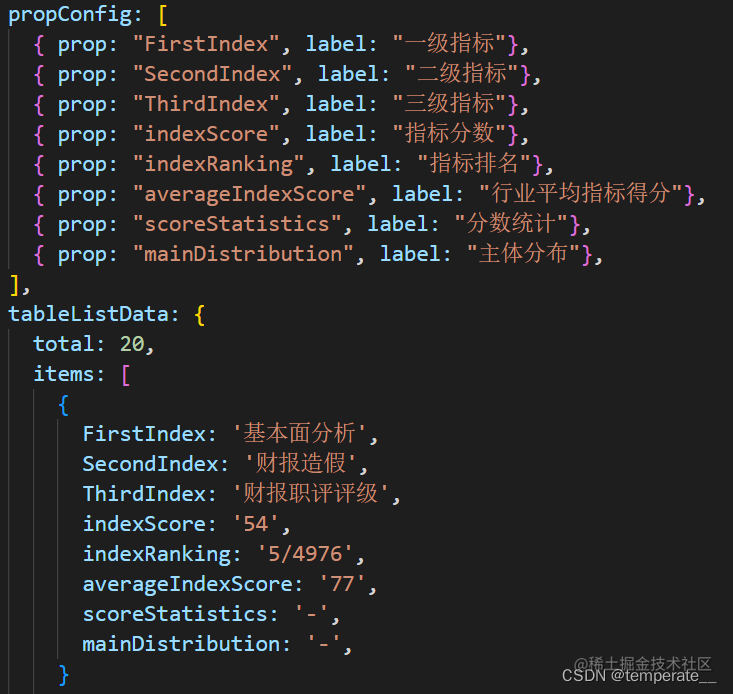## 处理数据

``````// data 为 表格数据 ， params 为需要合并的字段
groupBy (data, params) {const groups = {};
data.forEach(v => {// 获取data中的传入的params属性对应的属性值const group = JSON.stringify(v[params]);// 把group作为groups的key，初始化value，循环时找到相同的v[params]时不变
groups[group] = groups[group] || [];// 将对应找到的值作为value放入数组中
groups[group].push(v);
})// 返回处理好的二维数组return Object.values(groups);
},``````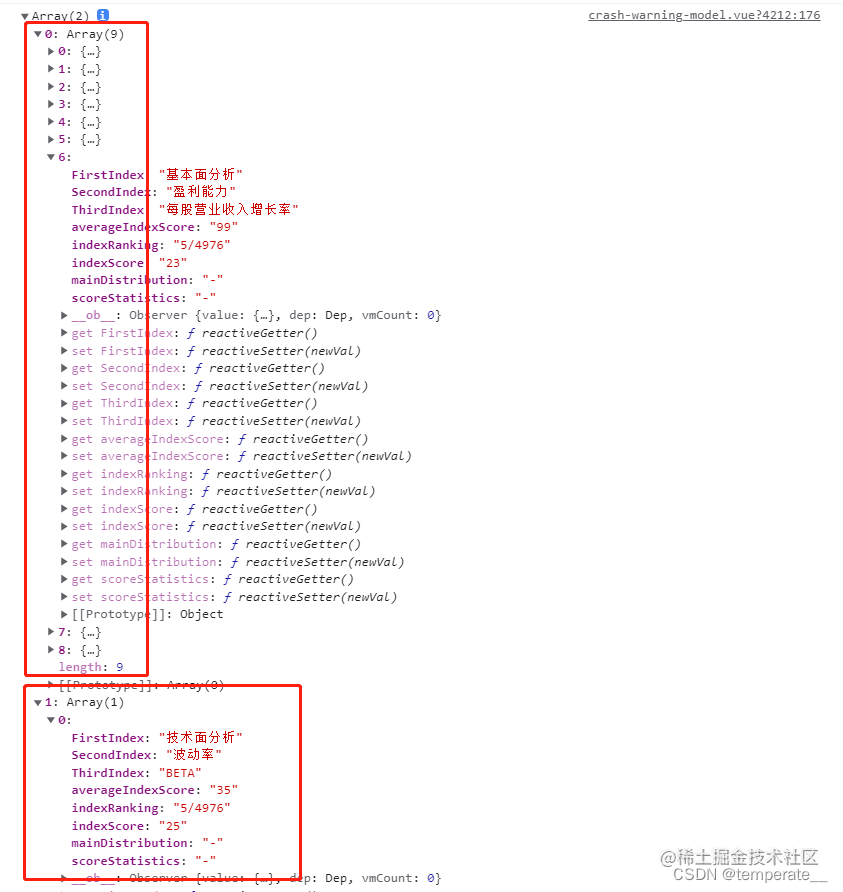## 构造控制合并的数组spanArr

• 接收重构数组 let arr = []
• 设置索引 let pos = 0
• 控制合并的数组 this.spanArr = []

`this.groupBy(this.tableListData.items, 'FirstIndex').map(v => (arr = arr.concat(v)))`

``````arr.map(res => {// 每次遍历都删除data && this.tableListData.items的第一个元素
data.shift()// 每次遍历都将arr数组元素对应push进 data && this.tableListData.items
data.push(res)
})``````

const redata = arr.map(v => v[params])

## reduce处理spanArr数组 ⭐⭐

``````  const redata = arr.map(v => v[params])
redata.reduce((old, cur, i) => {// old 上一个元素  cur 当前元素  i 索引if (i === 0) {// 第一次判断先增加一个 1 占位 ，索引为0 this.spanArr.push(1)
pos = 0
} else {if (cur === old) {this.spanArr[pos] += 1this.spanArr.push(0)
} else {this.spanArr.push(1)
pos = i
}
}return cur
}, {})``````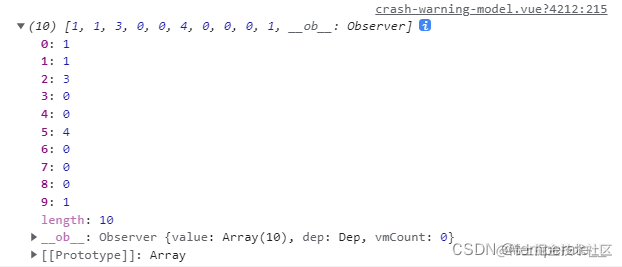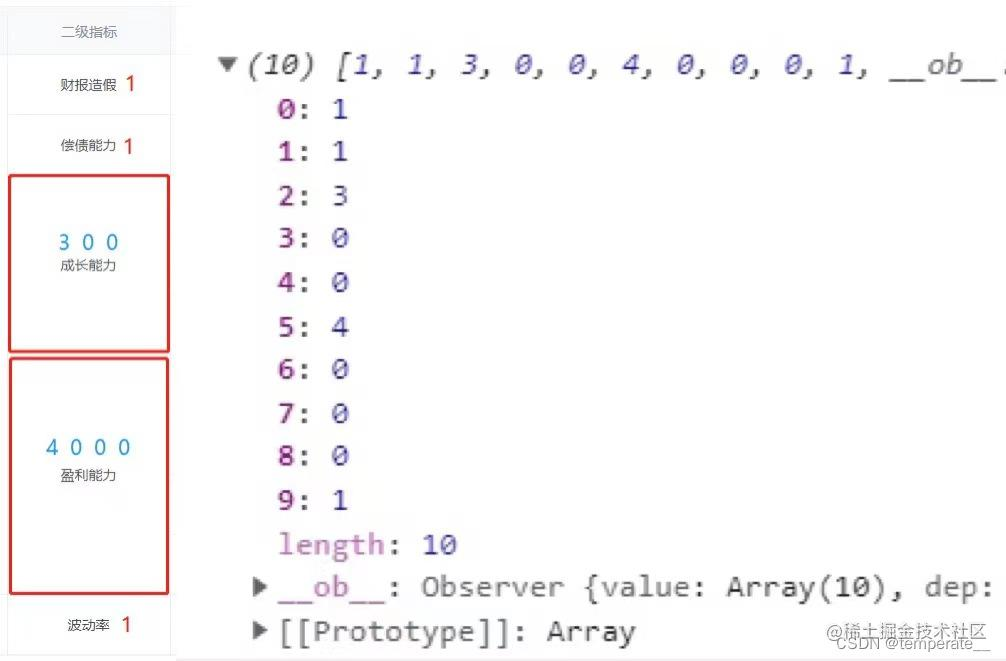## 返回最终结果

``````spanMethod({ row, column, rowIndex, columnIndex }) {// 第一列if (columnIndex === 0) {const _row = this.spanArr[rowIndex];const _col = _row > 0 ? 1 : 0;return {rowspan: _row,colspan: _col
}
}
}``````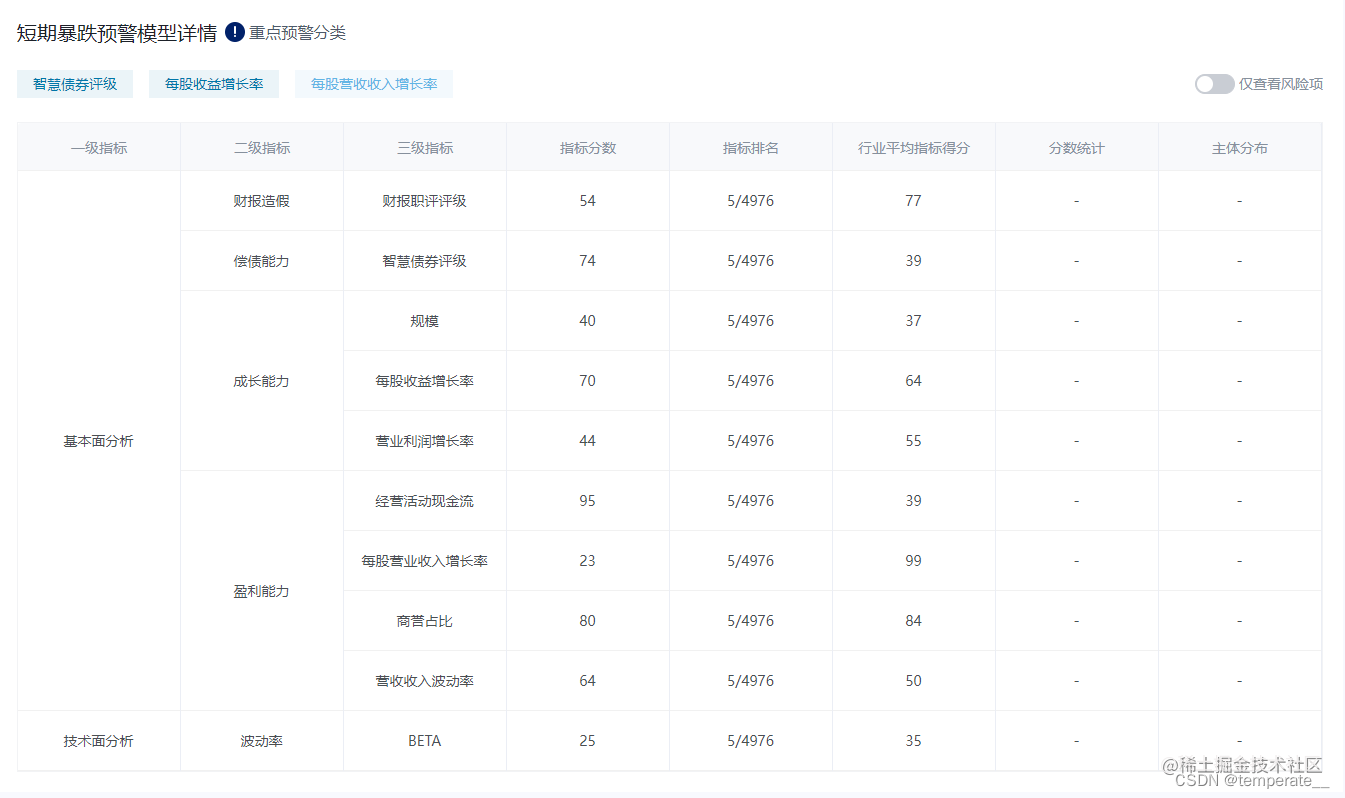## 完整代码

``````// ......mounted() {this.getSpanArr(this.tableListData.items, 'FirstIndex');
},methods: {
groupBy (data, params) {const groups = {}data.forEach(v => {const group = JSON.stringify(v[params])
groups[group] = groups[group] || []
groups[group].push(v)
})return Object.values(groups)
},
getSpanArr (data, params) {let arr = []let pos = 0this.spanArr = []this.groupBy(data, params).map(v => (arr = arr.concat(v)))
arr.map(res => {data.shift()data.push(res)
})const redata = arr.map(v => v[params])
redata.reduce((old, cur, i) => {if (i === 0) {this.spanArr.push(1)
pos = 0
} else {if (cur === old) {this.spanArr[pos] += 1this.spanArr.push(0)
} else {this.spanArr.push(1)
pos = i
}
}return cur
}, {})
},spanMethod({ row, column, rowIndex, columnIndex }) {if (columnIndex === 0) {const _row = this.spanArr[rowIndex];const _col = _row > 0 ? 1 : 0;return {rowspan: _row,colspan: _col
}
}
}
}``````